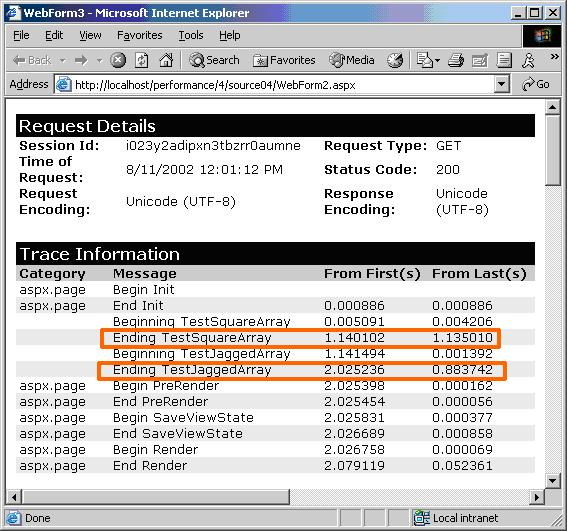# Jagged Arrays and Performance in .Net

Tuesday, September 21, 2004

There are three types of arrays you can use in .NET: single-dimensional arrays, multidimensional arrays, and jagged arrays. Jagged arrays, also known as arrays of arrays, offer not only a space savings in certain conditions, but also a performance enhancement, as we will demonstrate.

In this article, we are going to use a multidimensional array and a jagged array to hold random numbers for each day of the year. Two dimensions will allow us to reach any day of the year by providing the index of the month, and the index of the day. We declare the arrays using the syntax shown below.

`Private multi(11, 30) As IntegerPrivate jagged(11)() As Integer`

Notice for the multidimensional array we need to declare the second dimension of the array with the maximum number of days possible in a month (31). With two dimensions you can think of a multidimensional array as a square, since each row has the same number of columns. This leads to wasted space as not all of the array entries correspond to a valid day, for example, there is no 31st day in April.

Jagged arrays, on the other hand, allow you to allocate a different number of columns for each row. In the CreateArrays subroutine shown below, we populate both arrays inside of loops. Notice the jagged array initialization includes the extra step to allocate an array of days for each month. Using the exact amount of storage required for a month saves a small amount of memory in comparison to the multidimensional array. The savings to represent a calendar year as an array are small, but other applications might find more significant savings.

```Private Sub CreateArrays()  Dim i As Integer  Dim j As Integer  Dim random As New Random()
' initialize multi dimension array with random data for each day  For i = 0 To 11    For j = 0 To DateTime.DaysInMonth(DateTime.Now.Year, i + 1) - 1      multi(i, j) = random.Next(100)    Next  Next
' allocate and initialize jagged array with random data  For i = 0 To 11    jagged(i) = New Integer(DateTime.DaysInMonth(DateTime.Now.Year, i + 1) - 1) {}    For j = 0 To DateTime.DaysInMonth(DateTime.Now.Year, i + 1) - 1      jagged(i)(j) = random.Next(100)    Next
NextEnd Sub```

Obviously, a jagged array, when compared to a multidimensional array, might require slightly more time to create with the extra allocation steps inside the loop. However, you might be surprised to know a jagged array will generally outperform a multidimensional array when it comes time to access and iterate the arrays. In version 1 of the .NET framework, better optimizations exist in the JIT compiler for jagged arrays. To demonstrate, let’s look at the two test subroutines we run each time our test page executes.

```Private Sub TestSquareArray()  Trace.Write("Beginning TestSquareArray")
Dim i As Integer  Dim j As Integer  Dim k As Integer  Dim sum As Int64
sum = 0  For i = 0 To 65525    For j = 0 To 11      For k = 0 To DateTime.DaysInMonth(DateTime.Now.Year, j + 1) - 1        sum = sum + multi(j, k)      Next    Next  Next
Trace.Write("Ending TestSquareArray")End Sub
Private Sub TestJaggedArray()  Trace.Write("Beginning TestJaggedArray")
Dim i As Integer  Dim j As Integer  Dim k As Integer  Dim sum As Int64
sum = 0  For i = 0 To 65525    For j = 0 To 11      For k = 0 To DateTime.DaysInMonth(DateTime.Now.Year, j + 1) - 1        sum = sum + jagged(j)(k)      Next    Next  Next
Trace.Write("Ending TestJaggedArray")End Sub```

Each subroutine sums up the values inside of each array. We do this repeatedly inside of another large loop to lengthen the computation time and extract more meaningful timing information. After we enable tracing we can look at the timing information for the page, shown below. The routine accessing the jagged array is consistently around 20% faster than the multidimensional version.#### All My Pluralsight CoursesOdeToCode by K. Scott Allen